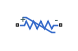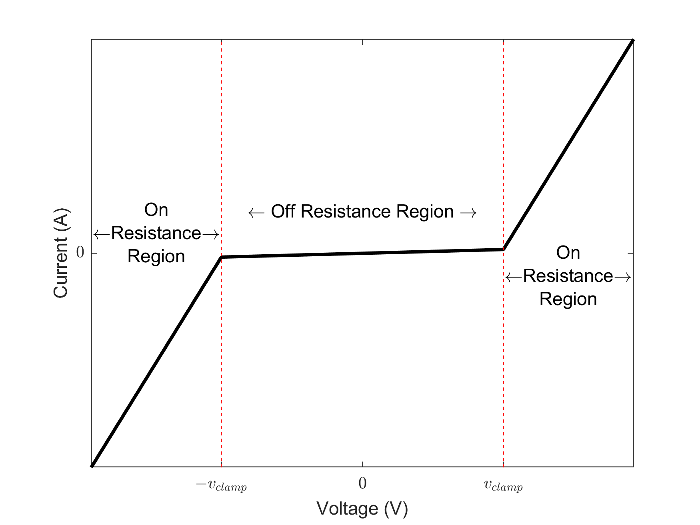# Varistor

Voltage-dependent resistor

• Library:
• Simscape / Electrical / Passive

•## Description

The Varistor block represents a voltage-dependent resistor (VDR). This component is also commonly known as a metal-oxide varistor (MOV). The block exhibits high resistance at low voltages and low resistance at high voltages.

You can protect parts of an electrical circuit from high-voltage surges by placing this block in parallel with them. When a surge occurs, the resistance of the varistor drops significantly, causing the current to be shunted through the varistor rather than through the circuit.

Use the Parameterization parameter to choose between two different behaviors for this block. The `Linear` option focuses on the on- and off-states of the varistor and uses a linear relationship between current and voltage in both regions. The `Power-law` option uses an exponential relationship between current and voltage in the initial on-state. This option also adds a third, linear region at higher voltages.

### Linear Parameterization

This parameterization option separates the voltage-current relationship into two linear regions:

• Off-region — resistance is high and current increases slowly with increasing voltage.

• On-region — resistance is low and current increases rapidly with increasing voltage.

This figure shows the voltage-current relationship across the on- and off-regions.Use linear parameterization in one of these scenarios:

• You are modeling voltage surges close to the threshold voltage

• You expect your varistor to behave linearly in all regions

The voltage-current relationship for the linear varistor is:

`${i}_{varistor}=\left\{\begin{array}{cc}\frac{{v}_{varistor}}{{R}_{off}}& |{v}_{varistor}|<{v}_{clamp}\\ \frac{{v}_{varistor}}{{R}_{on}}+{c}_{1}\mathrm{sgn}\left({v}_{varistor}\right)& |{v}_{varistor}|\ge {v}_{clamp}\end{array}.$`

where:

• vvaristor and ivaristor are the varistor voltage and current, respectively.

• vclamp is the threshold voltage that separates the two regions of operation. Set this value using the Clamping voltage parameter.

• Ron and Roff are the resistances in the on- and off-regions. Set these values using the On resistance and Off resistance, respectively.

• c1 is a constant used to enforce current continuity between the two regions:

`${c}_{1}={v}_{clamp}\left(\frac{1}{{R}_{off}}-\frac{1}{{R}_{on}}\right).$`

### Power-Law Parameterization

This parameterization option separates the voltage-current relationship into three regions:

• Leakage region — Resistance is high and current increases slowly with increasing voltage.

• Normal region — Resistance decreases exponentially with increasing voltage.

• Upturn region — Resistance is low and current increases rapidly with increasing voltage.

This figure shows the three regions of operation in log-log-scale.Use power-law parameterization in one of these scenarios:

• You are modeling voltage surges across a large range of voltages

• You expect your varistor to behave exponentially in the first on-region

The voltage-current relationship for the power-law varistor is:

`${i}_{varistor}=\left\{\begin{array}{cc}\frac{{v}_{varistor}}{{R}_{L}}& |{v}_{varistor}|<{v}_{LN}\\ k{\left({v}_{varistor}\right)}^{\alpha }+{c}_{1}& {v}_{LN}\le |{v}_{varistor}|\le {v}_{NU}\\ \frac{{v}_{varistor}}{{R}_{U}}+{c}_{2}& |{v}_{varistor}|>{v}_{NU}\end{array}$`

where:

• vvaristor and ivaristor are the varistor voltage and current, respectively.

• α is the power-law exponent which determines the rate of current increase with voltage increase in the normal region. Set this value using the Normal-mode power-law exponent parameter.

• vLN and the vNU are the threshold voltages corresponding to the leakage-normal and normal-upturn transition points. Set these values using the Leakage to normal voltage transition and Normal to upturn voltage transition parameters, respectively.

• RL and RU are the resistances in the leakage- and upturn-regions. Set these values using the Leakage-mode resistance and Upturn-mode resistance parameters, respectively.

• k, c1, and c2 are constants used to enforce current continuity between the regions:

`$k=\frac{1}{\alpha {R}_{U}{v}_{NU}^{\alpha -1}},$`

`${c}_{1}=\frac{{v}_{LN}}{{R}_{L}}-\frac{{v}_{LN}^{\alpha }}{\alpha {R}_{U}{v}_{NU}^{\alpha -1}},$`

and

`${c}_{2}=\frac{1}{\alpha {R}_{U}{v}_{NU}^{\alpha -1}}\left({v}_{NU}^{\alpha }-{v}_{LN}^{\alpha }\right)-\frac{{v}_{NU}}{{R}_{U}}+\frac{{v}_{LN}}{{R}_{L}}.$`

### Equivalent Circuit

In addition to the varistor equations, you can also specify a constant terminal resistance Rt and device capacitance C. This figure shows the equivalent circuit for the varistor in either parameterization mode.## Ports

### Conserving

expand all

Electrical conserving port associated with the varistor positive terminal.

Electrical conserving port associated with the varistor negative terminal.

## Parameters

expand all

Choose how the varistor resistance changes with increasing voltages:

• `Linear` — Two regions. The low-voltage region has high resistance and the high-voltage region has low resistance.

• `Power-law` — Three regions. The leakage region has high resistance. The normal region has exponentially decreasing resistance. The upturn region has low resistance.

Transition point voltage, vclamp, between the off- and on-states of the linear varistor.

#### Dependencies

Enabled when the Parameterization parameter is set to `Linear`.

Low-voltage resistance, Roff, of the varistor in the off-state.

#### Dependencies

Enabled when the Parameterization parameter is set to `Linear`.

High-voltage resistance, Ron, of the varistor in the on-state.

#### Dependencies

Enabled when the Parameterization parameter is set to `Linear`.

Transition point voltage, vLN, between the leakage and normal regions of the power-law varistor.

#### Dependencies

Enabled when the Parameterization parameter is set to `Power-law`.

Transition point voltage, vNU, between the normal and upturn regions of the power-law varistor.

#### Dependencies

Enabled when the Parameterization parameter is set to `Power-law`.

Low-voltage resistance, RL, of the varistor in the leakage region.

#### Dependencies

Enabled when the Parameterization parameter is set to `Power-law`.

Exponent that determines rate of current increase with voltage increase of the varistor in the normal region.

#### Dependencies

Enabled when the Parameterization parameter is set to `Power-law`.

High-voltage resistance, RU, of the varistor in the upturn region.

#### Dependencies

Enabled when the Parameterization parameter is set to `Power-law`.

Small, constant resistance in series with the varistor. Set this value to zero to remove the resistance from the equivalent circuit.

Capacitor in parallel with the varistor. Set this value to zero to remove the capacitor from the equivalent circuit.

## Version History

Introduced in R2018a